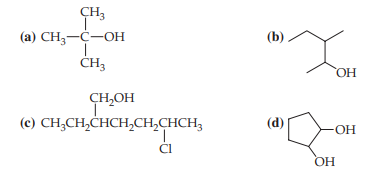×
Get Full Access to Fundamentals Of General, Organic, And Biological Chemistry (Mastering Chemistry) - 8 Edition - Chapter 14.2 - Problem 14.4
Get Full Access to Fundamentals Of General, Organic, And Biological Chemistry (Mastering Chemistry) - 8 Edition - Chapter 14.2 - Problem 14.4

×ISBN: 9780134015187 2044

## Solution for problem 14.4 Chapter 14.2

Fundamentals of General, Organic, and Biological Chemistry (Mastering Chemistry) | 8th Edition

• Textbook Solutions
• 2901 Step-by-step solutions solved by professors and subject experts
• Get 24/7 help from StudySoup virtual teaching assistantsFundamentals of General, Organic, and Biological Chemistry (Mastering Chemistry) | 8th Edition

4 5 1 391 Reviews
19
4
Problem 14.4

Give systematic names for the following compounds:Step-by-Step Solution:

Chapter 5 Energy  Energy- "something" an object has if it can do work  Work- force x distance  Kinetic energy- energy of motion KE= 1/2 mv^2  Potential energy- stored energy PE= mgh  Chemical energy- PE stored in compounds w. chemical bonds Units:  Calorie- amount of heat needed to raise the temperature of 1.00 g of water by 1 degree  1 nutritional "Calorie" = 1000 cal = 1 kilocalorie = 1 kcal  Joule- mass x velocity (kg m^2/ sec^2)  4.184 J = 1.00 cal Kinds of energy:  PE increases when objects that attract are separated/ objects that repel are moved closer together  Exothermic- process gives off energy (heat out)  Forming a chemical bond (negative sign dH)  Endothermic- process consumes energy ( heat in)  Breaking a chemical bond (positive sign dH) Reactions:  Thermite Reaction (exothermic) 2Al+ Fe O --> 2 3 Al 2 +3Fe (l)  Step 1 Iron oxide --> 2 Fe + 3O (bonds break= endothermic) 

Step 2 of 2

##### ISBN: 9780134015187

The full step-by-step solution to problem: 14.4 from chapter: 14.2 was answered by Aimee Notetaker, our top Chemistry solution expert on 04/25/22, 03:57PM. This textbook survival guide was created for the textbook: Fundamentals of General, Organic, and Biological Chemistry (Mastering Chemistry), edition: 8. This full solution covers the following key subjects: . This expansive textbook survival guide covers 281 chapters, and 456 solutions. The answer to “?Give systematic names for the following compounds:” is broken down into a number of easy to follow steps, and 7 words. Since the solution to 14.4 from 14.2 chapter was answered, more than 201 students have viewed the full step-by-step answer. Fundamentals of General, Organic, and Biological Chemistry (Mastering Chemistry) was written by Aimee Notetaker and is associated to the ISBN: 9780134015187.

## Discover and learn what students are asking

Statistics: Informed Decisions Using Data : Testing the Significance of the Least-Squares Regression Model
?Why is it important to perform graphical as well as analytical analyses when analyzing relations between two quantitative variables?

Unlock Textbook Solution